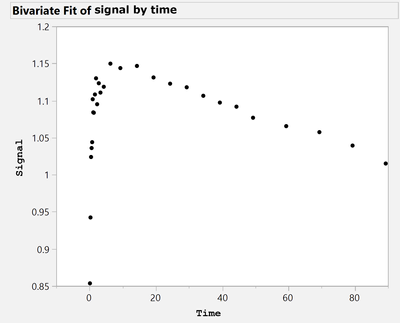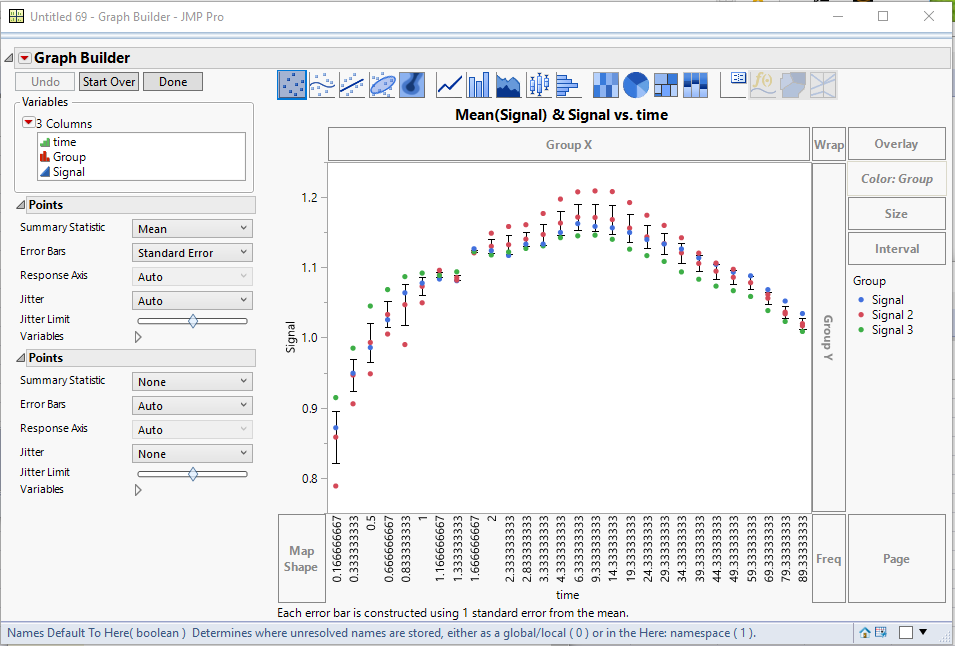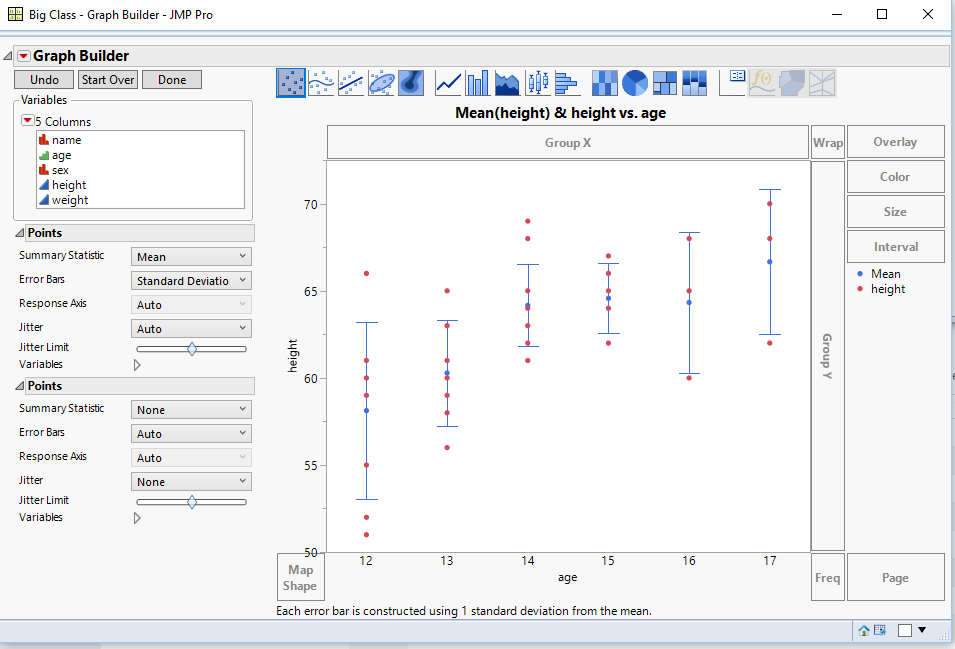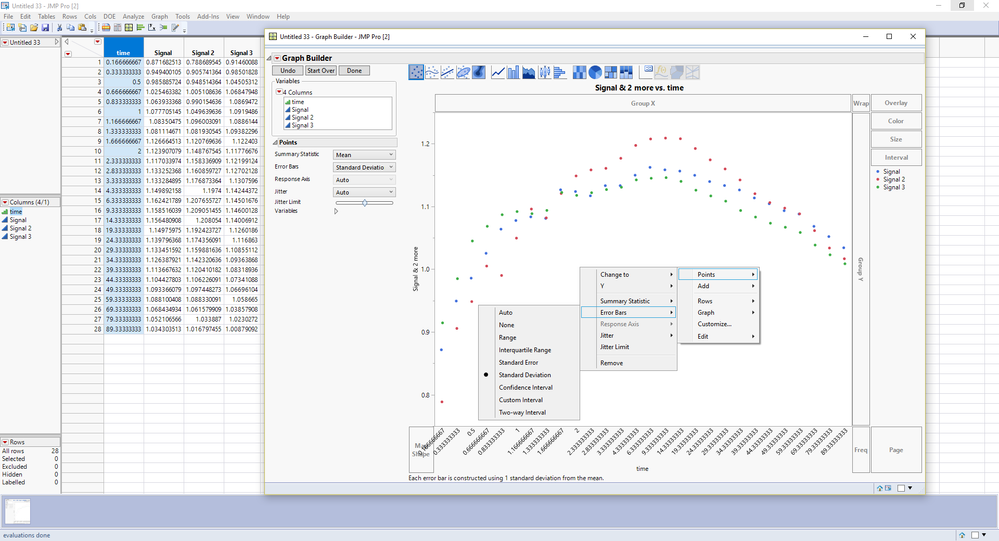Choose Language Hide Translation Bar
Highlighted

## Standard deviation Bars

Hello,

I would like to plot Y by X continous variables. X represent unit time., and Y is mean signal at each time point in X (please see below). I would like to add standard deviation bars to each point. How can I do it in JMP pro 14?john

John anderson
1 ACCEPTED SOLUTION

Accepted Solutions
Highlighted

## Re: Standard deviation Bars

Here is the graphI Stacked the columns

Tables==>Stack

Choosing the 3 Signal columns, Selecting to Keep the Time column, and changing the names of the output columns from Label to Group and Data to Signal

Then using Graph Builder, I dragged Signal to Y, Time to X.  I changed the Summary Statistic to Mean and then set the Error Bars to Standard Error.

Next I Right Clicked on the graph and selected Add==>Points

Below is a script that performs these tasks in JSL

``````Names Default To Here( 1 );
dt = Data Table( "jmp_signals" );
dtStack = dt <<
Stack(
columns( :Signal 2, :Signal 3, :Signal ),
Source Label Column( "Group" ),
Stacked Data Column( "Signal" )
);

dtStack << Graph Builder(
Variables( X( :time ), Y( :Signal ), Color( :Group ) ),
Elements(
Points(
X,
Y,
Legend( 10 ),
Summary Statistic( "Mean" ),
Error Bars( "Standard Error" )
),
Points( X, Y, Legend( 11 ), Jitter( "None" ) )
)
);``````
Jim
11 REPLIES 11
Highlighted

## Re: Standard deviation Bars

You can easily handle this using Graph BuilderJim
Highlighted

## Re: Standard deviation Bars

Dear txnelson – thank you so much for the response! I think you were able to show the standard deviation bars because the modeling type for X (i.e. age) is ordinal not continous. Correct? Is there any differnce?

Kindly, how happened that you have multiple heights for each age group. I expect that your spreadsheet has three columns one for age, the other one for height, and the third one for weight?

Also the title of your figure was "mean (height) & height vs age". That means you ploted two Y variables against one X variable (i.e. age). Correct?

I coud not do it! In my spreadsheet I have only two columns one for X and the other one for Y. both are continous variables. I highly appreciate any guidance. Thanks!

John anderson
Highlighted

## Re: Standard deviation Bars

Where are you expecting that you are going to get your standard deviation?  If you X variable is continuious.....each individual point will be considered independently.

You can easily change the Modeling Type from Continuous to Ordinal, if you have multiple values at the differenx X's

Jim
Highlighted

## Re: Standard deviation Bars

Dear txnelson – I tried to change X to ordinal. When ploting X vs Y this will output one point in X for each point in Y. In the figure you provided there are multiple points in Y for each X. Kindly, why?

John anderson
Highlighted

## Re: Standard deviation Bars

Just add a new Points entry for the chart.

Right click on the chart, and select "Add" and then select "Points"

Jim
Highlighted

## Re: Standard deviation Bars

Kindly see bellow is my spreadsheet and the graph builder which failed to show the "Standard deviation Bars". I am not sure what I am doing wrong?!John anderson
Highlighted

## Re: Standard deviation Bars

You have separated your y column into 3 separate columns, which will not allow Graph Builder to group them for the calculation of the standard deviation.

If you Stack the 3 Signal columns, keeping the Time column too, then you can drag the stacked column to Y and Time to X, Color by the Label column from the Stack output, and then ask for the Error Bars, and add the new Points.

If this is too confusing, attach your data table, an I will take a go at it

Jim
Highlighted

## Re: Standard deviation Bars

Dear txnelson please see attached is the spreadsheet. Thank you so much for the help!

John anderson
Highlighted

## Re: Standard deviation Bars

Here is the graphI Stacked the columns

Tables==>Stack

Choosing the 3 Signal columns, Selecting to Keep the Time column, and changing the names of the output columns from Label to Group and Data to Signal

Then using Graph Builder, I dragged Signal to Y, Time to X.  I changed the Summary Statistic to Mean and then set the Error Bars to Standard Error.

Next I Right Clicked on the graph and selected Add==>Points

Below is a script that performs these tasks in JSL

``````Names Default To Here( 1 );
dt = Data Table( "jmp_signals" );
dtStack = dt <<
Stack(
columns( :Signal 2, :Signal 3, :Signal ),
Source Label Column( "Group" ),
Stacked Data Column( "Signal" )
);

dtStack << Graph Builder(
Variables( X( :time ), Y( :Signal ), Color( :Group ) ),
Elements(
Points(
X,
Y,
Legend( 10 ),
Summary Statistic( "Mean" ),
Error Bars( "Standard Error" )
),
Points( X, Y, Legend( 11 ), Jitter( "None" ) )
)
);``````
Jim
Article Labels

There are no labels assigned to this post.